• Call Now

1800-102-2727•

# Electric Dipole: Definition, SI Unit, Formula, Dipole Moment

The behaviour of the dipoles of different materials gives rise to interesting properties and thus we can derive important applications based on them. Study of electric dipoles helps us to understand the applications and behaviour of polar molecules having permanent electrical moment even in the absence of electric field. In most of the molecules, the centre of positive charge and centre of negative charge coincide. So, their dipole moment is zero. When an electric field is applied the centre of positive charge and centre of negative charge will shift giving rise to the dipole moment. Some molecules like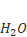have permanent dipole moments even in the absence of an electrical field.

Table of content:

• Electric Dipole
• Dipole Moment
• Practice Problems
• FAQs

## Electric Dipole:

The electric dipole is a system of two point-charges which are equal in magnitude but opposite in nature. These point charges are separated by somedistance.

The midpoint of location of –and is called the centre of the dipole.

The axis of the dipole is the line joining the negative and positive charges.

The equatorial line is the perpendicular bisector of the axis of the dipole.

The plane, perpendicular to the dipole axis and passes through the equatorial line of the dipole is known as the equatorial plane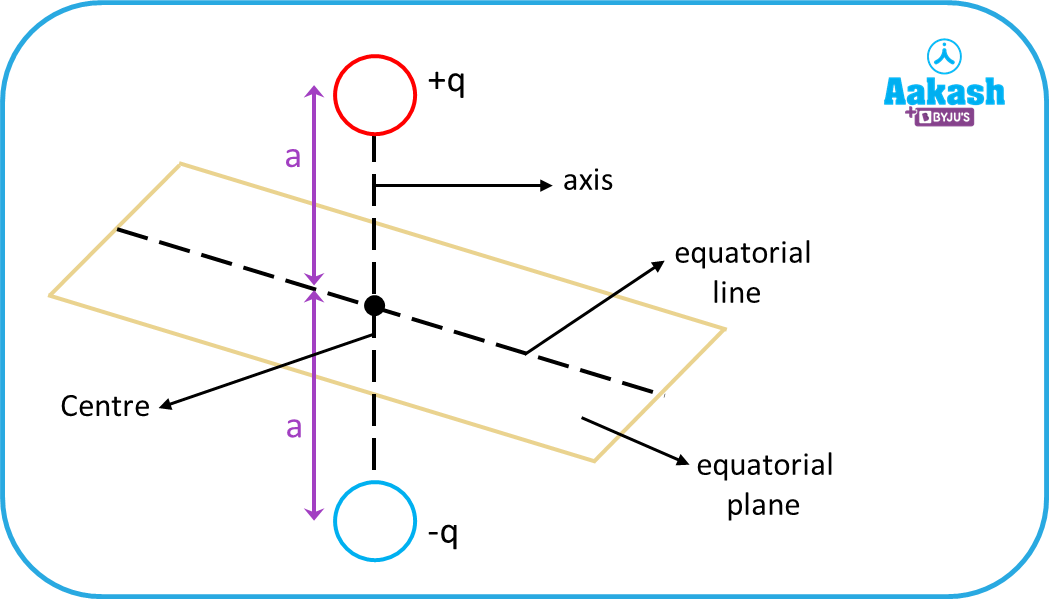## Dipole Moment:

The dipole moment of the electric dipole is a vector quantity.

Magnitude of the dipole moment is the product of the magnitude of either of the charges and the separation distance between them. If the magnitude of the charges is q and the separation distance between them is 2a, then the magnitude of the dipole moment is defined as = 2aq

Direction is along the axis of the dipole (directed from the negative charge to positive charge).

SI unit of the electric dipole moment is Cm

Electric dipoles need not necessarily be only two point-charge systems which are equal in magnitude and opposite in nature. An electric dipole is an electrically neutral system and the smallest possible case of an electrically neutral system is that of one positive and one negative charge. It can also be a system of charges but it should be neutral.

An electrically neutral system consisting of equal numbers of positive and negative charges also forms a dipole, if the centre of the positive charges, which is known as the centre of positivity (like the COM of many discrete particles), and the centre of negative charges, which is known as the centre of negativity, do not coincide with each other, i.e., they are separated by some distance.

The centre of positivity or negativity can be derived the same way as the centre of mass. For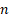number of positive (or negative) charges the centre of positivity(or negativity) can be calculated as,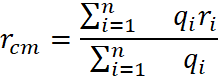This is the reason an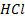molecule has an electric dipole moment.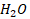molecule also has an electric dipole moment. However, an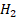molecule does not have an electric dipole moment because for themolecule, due to symmetry (linear molecule with the same atom at both the sides of the bond), the centre of the positive charge and the centre of the negative charge coincide with each other. Same with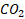and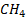molecule. They have zero dipole moment.

Note: An electrically neutral system has the possibility of becoming an electric dipole. To have a non-zero electric dipole moment, the centre of the positive charges (centre of positivity) and the centre of negative charges (centre of negativity) of the system must be separated by some distance.

## Practice Problems:

Q. Two point-charges of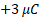and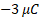are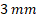apart. What will be the dipole moment for the system?

A. The dipole moment for a two point-charge system is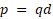, where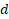is the separation between them.

Here,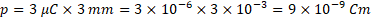Q. Find the electric dipole moment of the equilateral triangle of side length d formed by three charges as shown in the figure.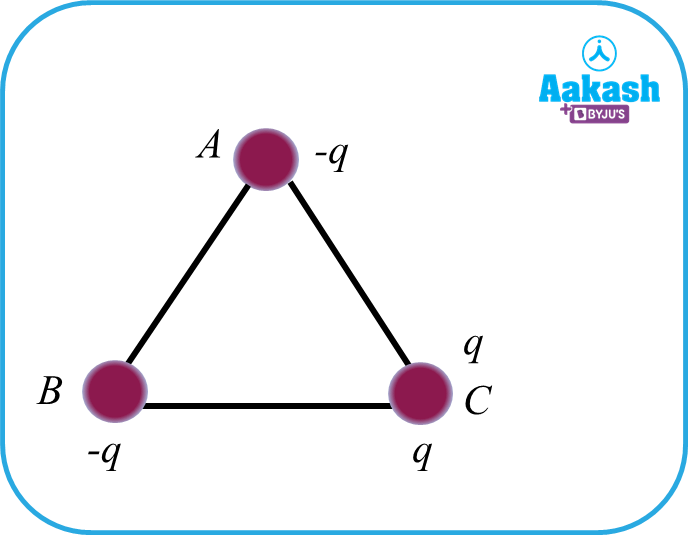A. The two negative charges are concentrated at the centre of the line joining them and the charge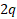remains at C. So, it forms an electric dipole consisting of chargesand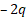.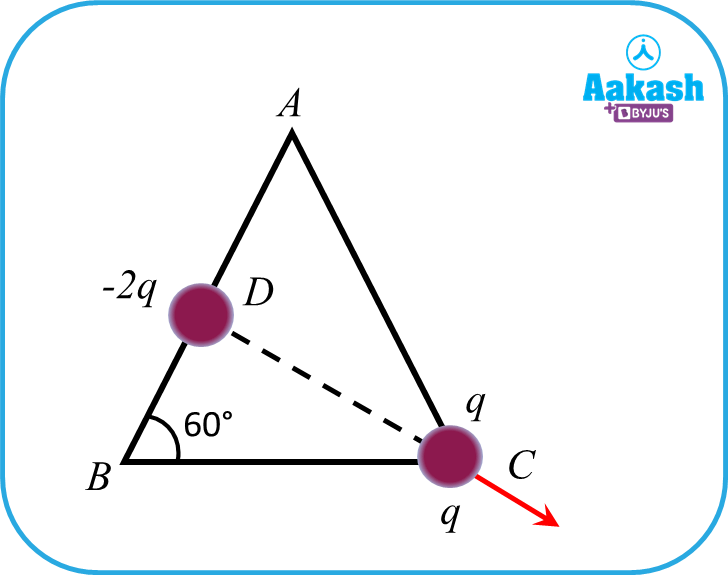The length of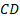is equal to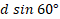.

Therefore, the dipole moment is given by,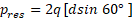The direction of the dipole moment will be along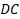.

Q. Find the electric dipole moment of the equilateral triangle formed by three charges as shown in the figure.A. The two positive charges are concentrated at the point D of the line joining them and the charge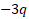remains at C. So, it forms an electric dipole consisting of charges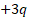and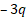.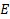is the mid-point on the line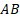.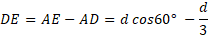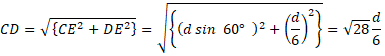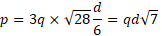Q. An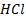molecule has a dipole moment of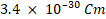. Assume equal and opposite charges lie on the two atoms forming the dipole. What will be the magnitude of the charge? [The separation distance between the two atoms ofis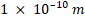]

A. The dipole moment is given by the formula,, whereis the separation between the positive and the negative charges and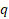is the magnitude of the charges.

The distance between the charges,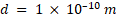The dipole moment,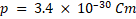Therefore,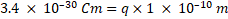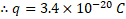## FAQs:

Q. Which of the following molecules have a dipole moment?

a.b.c.b.A.Only themolecule has the polarity i.e. a centre of positive and centre of negative charge are separated by some distance.

Q. Electric dipole is a

a. electrically charged system

b. electrically neutral system

c. only two point-charges system

b. none of these

A. electrically neutral system

Electric dipole is created by at least two charges of equal magnitude but opposite in nature.

Q. Which of the combinations may constitute an electric dipole?

a.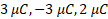b.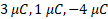c.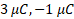d. none of these

A.Electric dipole is neutral in nature. So their net charge (sum of all the charges) is zero.

Q. Two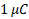point charges are placed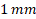apart. The dipole moment created is,

a.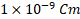b.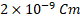c.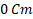d. none of these

A.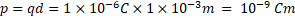Talk to our expert
Resend OTP Timer =
By submitting up, I agree to receive all the Whatsapp communication on my registered number and Aakash terms and conditions and privacy policy